To prove one-one & onto (injective, surjective, bijective)

Chapter 1 Class 12 Relation and Functions
Concept wise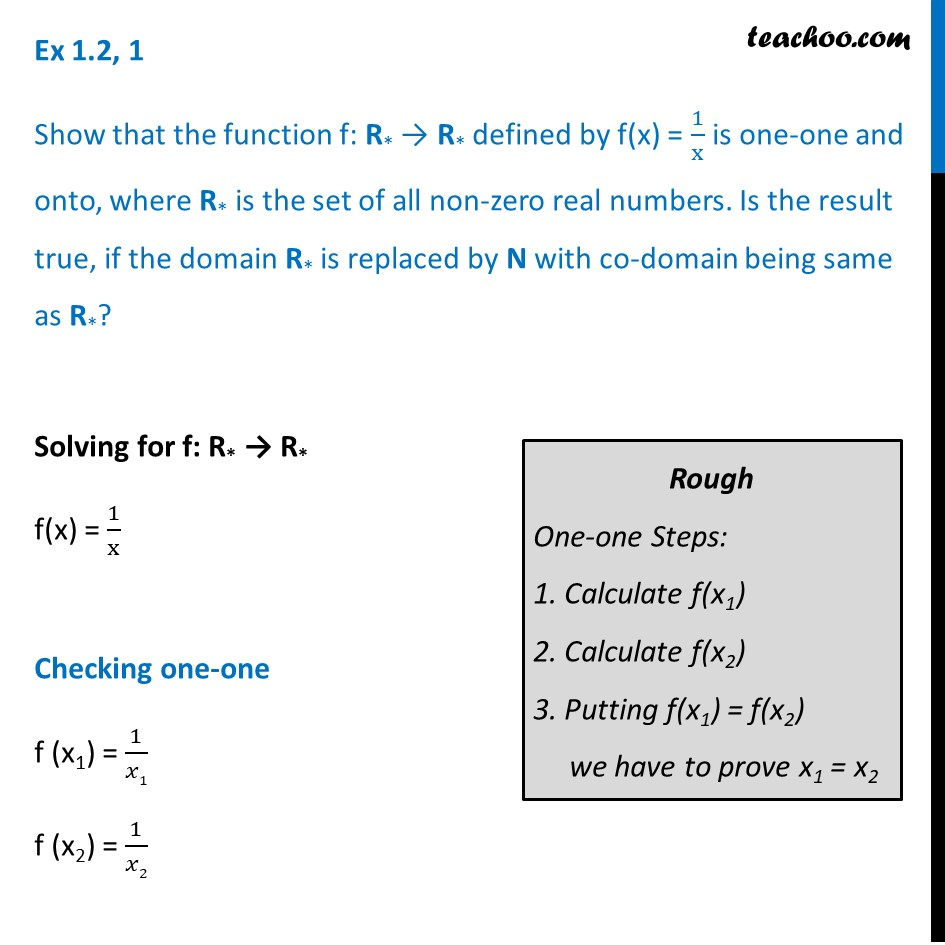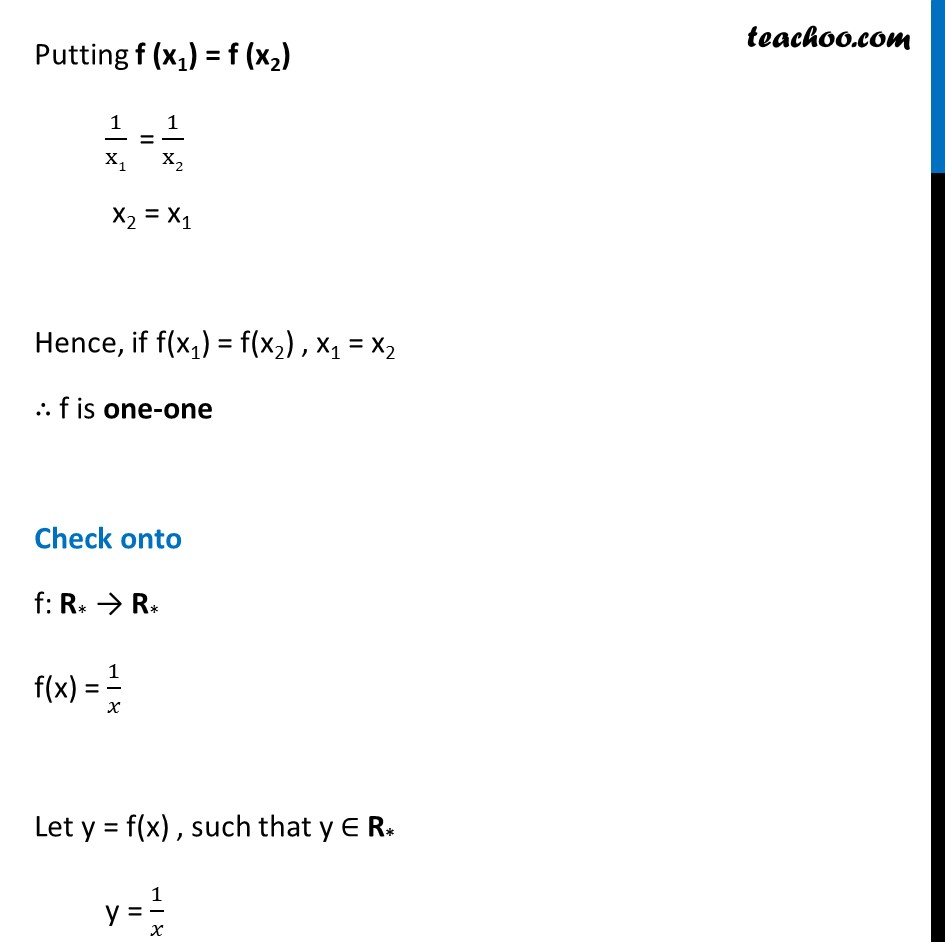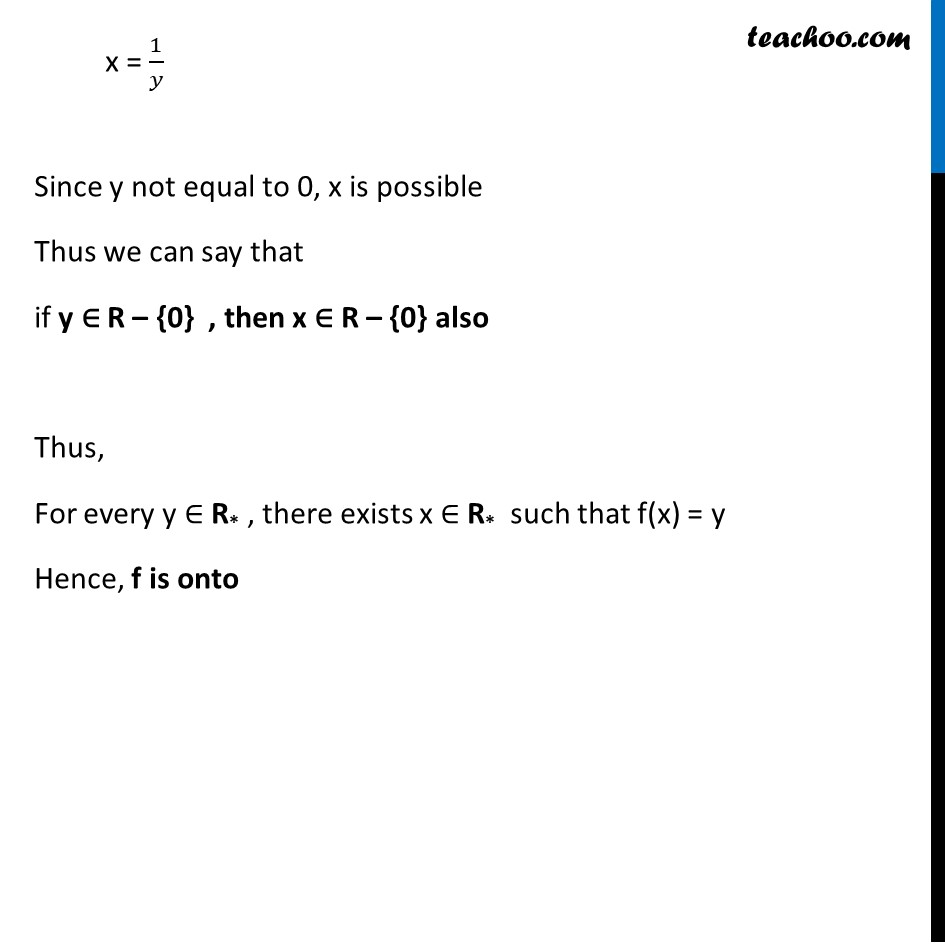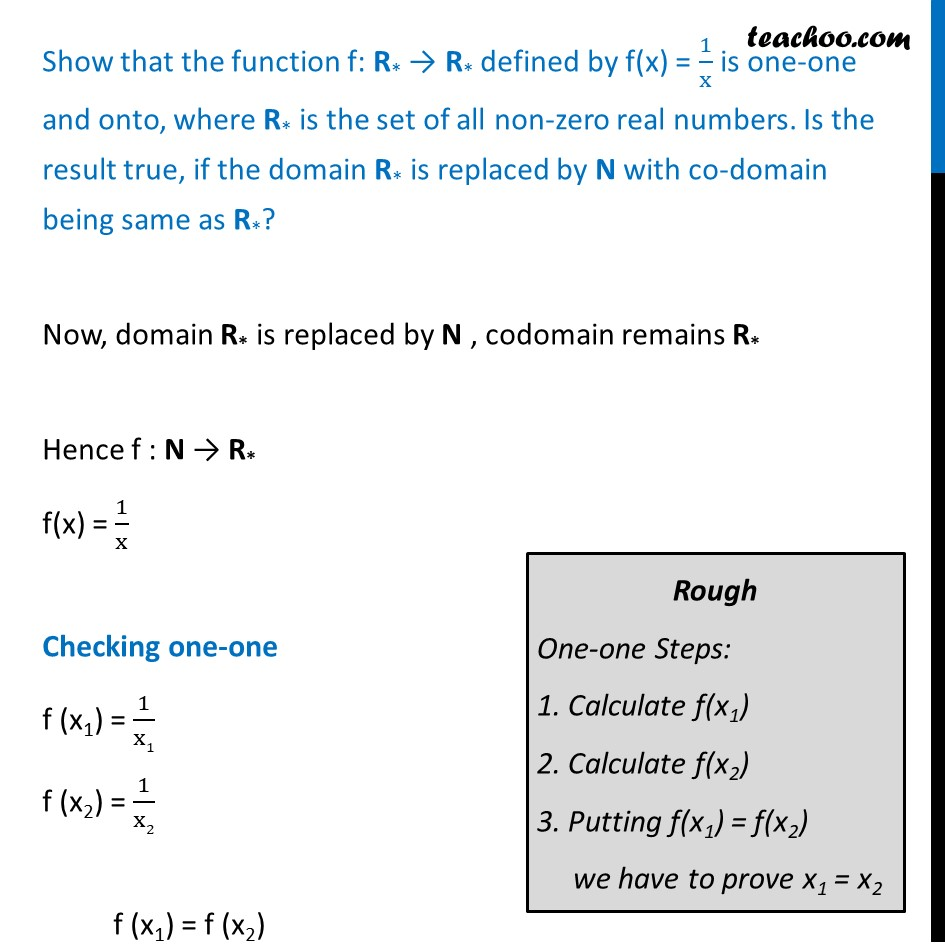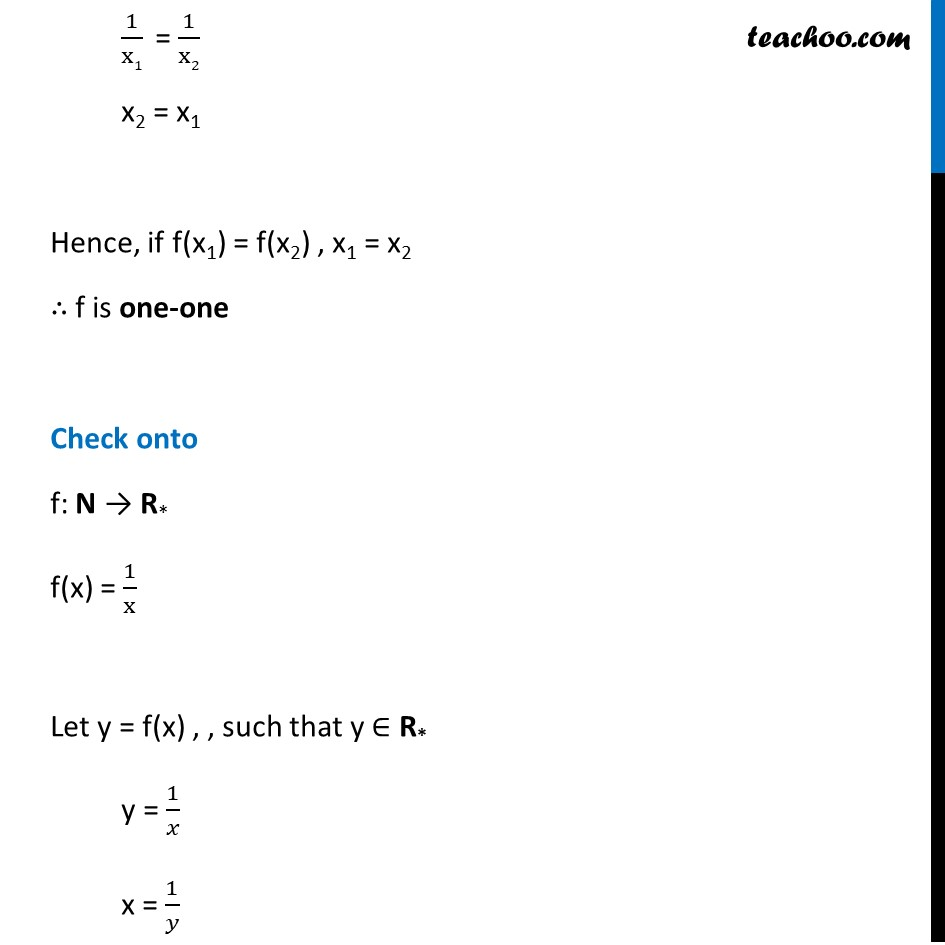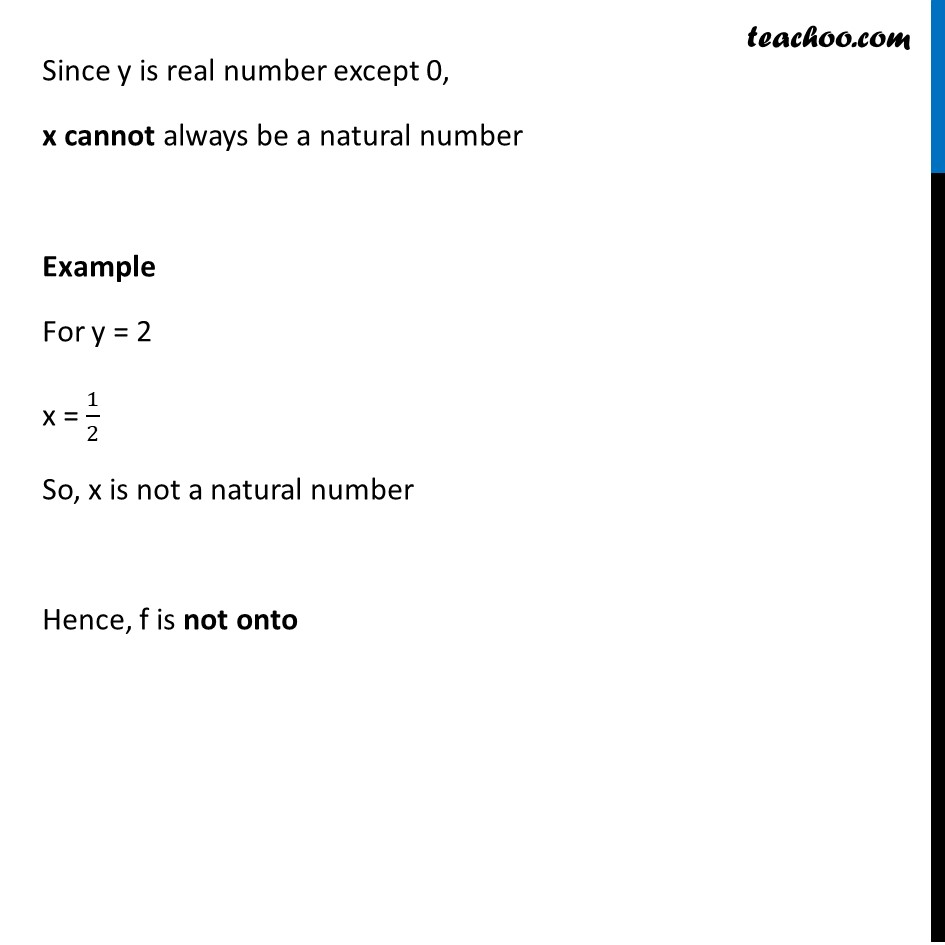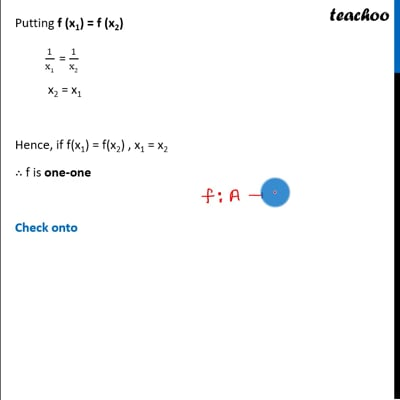This video is only available for Teachoo black usersThis video is only available for Teachoo black users

Learn in your speed, with individual attention - Teachoo Maths 1-on-1 Class

### Transcript

Ex 1.2, 1 Show that the function f: R* → R* defined by f(x) = 1/x is one-one and onto, where R* is the set of all non-zero real numbers. Is the result true, if the domain R* is replaced by N with co-domain being same as R*? Solving for f: R* → R* f(x) = 1/x Checking one-one f (x1) = 1/𝑥1 f (x2) = 1/𝑥2 Putting f (x1) = f (x2) 1/x1 = 1/x2 x2 = x1 Hence, if f(x1) = f(x2) , x1 = x2 ∴ f is one-one Check onto f: R* → R* f(x) = 1/𝑥 Let y = f(x) , such that y ∈ R* y = 1/𝑥 x = 1/𝑦 Since y not equal to 0, x is possible Thus we can say that if y ∈ R – {0} , then x ∈ R – {0} also Thus, For every y ∈ R* , there exists x ∈ R* such that f(x) = y Hence, f is onto Show that the function f: R* → R* defined by f(x) = 1/x is one-one and onto, where R* is the set of all non-zero real numbers. Is the result true, if the domain R* is replaced by N with co-domain being same as R*? Now, domain R* is replaced by N , codomain remains R* Hence f : N → R* f(x) = 1/x Checking one-one f (x1) = 1/x1 f (x2) = 1/x2 f (x1) = f (x2) 1/x1 = 1/x2 x2 = x1 Hence, if f(x1) = f(x2) , x1 = x2 ∴ f is one-one Check onto f: N → R* f(x) = 1/x Let y = f(x) , , such that y ∈ R* y = 1/𝑥 x = 1/𝑦Since y is real number except 0, x cannot always be a natural number Example For y = 2 x = 1/2 So, x is not a natural number Hence, f is not onto